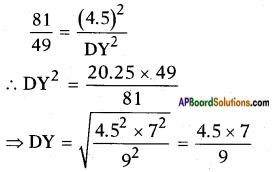# AP SSC 10th Class Maths Solutions Chapter 8 Similar Triangles Ex 8.3

AP State Board Syllabus AP SSC 10th Class Maths Textbook Solutions Chapter 8 Similar Triangles Ex 8.3 Textbook Questions and Answers.

## AP State Syllabus SSC 10th Class Maths Solutions 8th Lesson Similar Triangles Exercise 8.3

### 10th Class Maths 8th Lesson Similar Triangles Ex 8.3 Textbook Questions and Answers

Question 1.
Equilateral triangles are drawn on the three sides of a right angled triangle. Show that the area of the triangle on the hypotenuse is equal to the sum of the areas of triangles on the other two sides.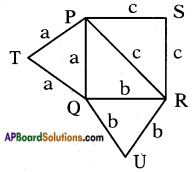Let △PQR is a right angled triangle, ∠Q = 90°
Let PQ = a, QR b and
PR = hypotenuse = c
Then from Pythagoras theorem we can
say a2 + b2 = c2 ……… (1)
△PSR is an equilateral triangle drawn on hypotenuse
∴ PR = PS = RS = c,
Then area of triangle on hypotenuse
= $$\frac{\sqrt{3}}{4}$$c2 ……… (2)
△QRU is an equilateral triangle drawn on the side ‘QR’ = b
∴ QR = RU = QU = b
Then area of equilateral triangle drawn on the side = $$\frac{\sqrt{3}}{4}$$b2 …….. (3)
△PQT is an equilateral triangle drawn on another side ‘PQ’ = a
∴ PQ = PT = QT = a
Area of an equilateral triangle drawn an another side ‘PQ’ = $$\frac{\sqrt{3}}{4}$$a2 …….. (4)
Now sum of areas of equilateral triangles on the other two sides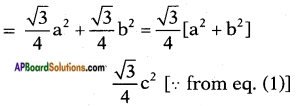= Area of equilateral triangle on the hypotenuse.
Hence Proved.Question 2.
Prove that the area of the equilateral triangle described on the side of a square is half the area of the equilateral triangles described on its diagonal.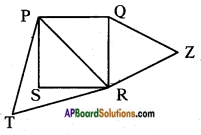Let PQRS is square whose side is ‘a’ units then PQ = QR = RS = SP = ‘a’ units.
Then the diagonal
$$\overline{\mathrm{PR}}$$ = $$\sqrt{a^{2}+a^{2}}$$ = a√2 units.
Let △PRT is an equilateral triangle, then PR = RT = PT = a√2
∴ Area of equilateral triangle constructed on diagonal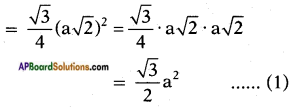Let △QRZ is another equilateral triangle whose sides are
$$\overline{\mathrm{QR}}$$ = $$\overline{\mathrm{RZ}}$$ = $$\overline{\mathrm{QZ}}$$ = ‘a’ units
Then the area of equilateral triangle constructed on one side of square = $$\frac{\sqrt{3}}{4}$$a2 ……. (2)
∴ $$\frac{1}{2}$$ of area of equilateral triangle on diagonal = $$\frac{1}{2}\left(\frac{\sqrt{3}}{2} a^{2}\right)$$ = $$\frac{\sqrt{3}}{4}$$a2 = area of equilateral triangle on the side of square.
Hence Proved.

Question 3.
D, E, F are midpoints of sides BC, CA, AB of △ABC. Find the ratio of areas of △DEF and △ABC.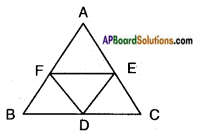Given in △ABC D, E, F are the midpoints of the sides BC, CA and AB.
In △ABC, EF is the line join of mid-points of two sides AB and AC of △ABC.
Thus FE || BC [∵ $$\frac{AF}{FB}$$ = $$\frac{AE}{EC}$$ Converse of B.P.T.]
Similarly DE divides AC and BC in the same ratio, i.e., DE || AB.
Now in □ BDEF, both pairs of opposite sides (BD || EF and DE || BF) are parallel.
Hence □ BDEF is a parallelogram where DF is a diagonal.
∴ △BDF ≃ △DEF ……… (1)
Similarly we can prove that
△DEF ≃ △CDE ……… (2)
[∵ CDFE is a parallelogram]
Also, △DEF ≃ △AEF …….. (3)
[∵ □ AEDF is a parallelogram]
From (1), (2) and (3)
△AEF ≃ △DEF ≃ BDF ≃ △CDE
Also, △ABC = △AEF + △DEF + △BDF + △CDE = 4 . △DEF
Hence, △ABC : △DEF = 4 : 1.Question 4.
In △ABC, XY || AC and XY divides the triangle into two parts of equal area. Find the ratio of $$\frac{AX}{XB}$$.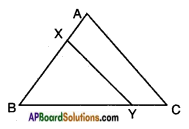Given: In △ABC, XY || AC.
XY divides △ABC into two points of equal area.
In △ABC, △XBY
∠B = ∠B
∠A = ∠X
∠C = ∠Y
[∵ XY || AC; (∠A, ∠X) and ∠C, ∠Y are the pairs of corresponding angles]
Thus △ABC ~ △XBY by A.A.A similarity condition.
Hence $$\frac{\Delta \mathrm{ABC}}{\Delta \mathrm{XBY}}=\frac{\mathrm{AB}^{2}}{\mathrm{XB}^{2}}$$
[∵ The ratio of areas of two similar triangles is equal to the ratio of squares of their corresponding sides]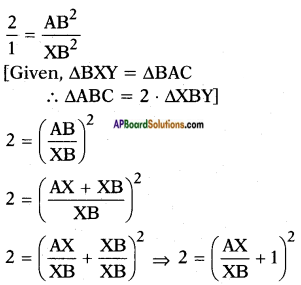⇒ $$\frac{AX}{XB}$$ + 1 = √2
⇒ $$\frac{AX}{XB}$$ = √2 – 1
Hence the ratio $$\frac{AX}{XB}$$ = $$\frac{√2 – 1}{1}$$.

Question 5.
Prove that the ratio of areas of two similar triangles is equal to the square of the ratio of their corresponding medians.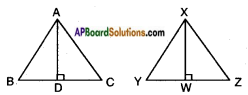Given: △ABC ~ △XYZ
R.T.P: $$\frac{\Delta \mathrm{ABC}}{\Delta \mathrm{XYZ}}=\frac{\mathrm{AD}^{2}}{\mathrm{XW}^{2}}$$
Proof : We know that the ratio of areas of two similar triangles is equal to the ratio of the squares of their corresponding sides.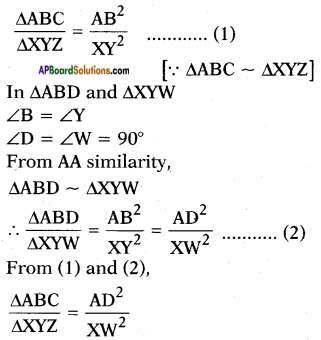Hence the ratio of areas of two similar triangles is equal to the squares of ratio of their corresponding medians.Question 6.
△ABC ~ △DEF. BC = 3 cm, EF = 4 cm and area of △ABC = 54 cm2. Determine the area of △DEF.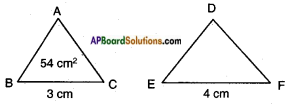Given: △ABC ~ △DEF
BC = 3 cm
EF = 4 cm
△ABC = 54 cm2
∴ △ABC ~ △DEF, we have
$$\frac{\Delta \mathrm{ABC}}{\Delta \mathrm{DEF}}=\frac{\mathrm{BC}^{2}}{\mathrm{EF}^{2}}$$
[∵ The ratio of two similar triangles is equal to the ratio of the squares of the corresponding sides].
$$\frac{54}{\Delta \mathrm{DEF}}$$ = $$\frac{3^{2}}{4^{2}}$$
∴ △DEF = $$\frac{54 \times 16}{9}$$ = 96 cm2

Question 7.
ABC is a triangle and PQ is a straight line meeting AB in P and AC in Q. If AP = 1 cm and BP = 3 cm, AQ =1.5 cm, CQ = 4.5 cm. Prove that area of △APQ = $$\frac{1}{16}$$ (area of △ABC).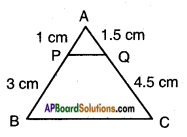Given: △ABC and $$\overline{\mathrm{PQ}}$$ – a line segment meeting AB in P and AC in Q.
AP = 1 cm; AQ =1.5 cm;
BP = 3 cm; CQ = 4.5 cm.
$$\frac{AP}{PQ}$$ = $$\frac{1}{3}$$ ……… (1)
$$\frac{AQ}{QC}$$ = $$\frac{1.5}{4.5}$$ = $$\frac{1}{3}$$ ……..(2)
From (1) and (2),
$$\frac{AP}{BP}$$ = $$\frac{AQ}{CQ}$$
[i.e., PQ divides AB and AC in the same ratio – By converse of Basic pro-portionality theorem]
Hence, PQ || BC.
Now in △APQ and △ABC
∠A = ∠A (Common)
∠P = ∠B [∵ Corresponding angles for the parallel lines PQ and BC]
∠Q = ∠C
∴ △APQ ~ △ABC [∵ A.A.A similarity condition]
Now, $$\frac{\Delta \mathrm{APQ}}{\Delta \mathrm{ABC}}=\frac{\mathrm{AP}^{2}}{\mathrm{AB}^{2}}$$
[∵ Ratio of two similar triangles is equal to the ratio of the squares of their corresponding sides].
= $$\frac{1^{2}}{(3+1)^{2}}$$ = $$\frac{1}{16}$$ [∵ AB = AP + BP = 1 + 3 = 4 cm]
∴ △APQ = $$\frac{1}{16}$$ (area of △ABC) [Q.E.D]

Question 8.
The areas of two similar triangles are 81 cm2 and 49 cm2 respectively. If the altitude of the bigger triangle is 4.5 cm. Find the corresponding altitude of the smaller triangle.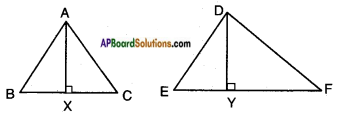$$\frac{\Delta \mathrm{ABC}}{\Delta \mathrm{DEF}}=\frac{\mathrm{AX}^{2}}{\mathrm{DY}^{2}}$$